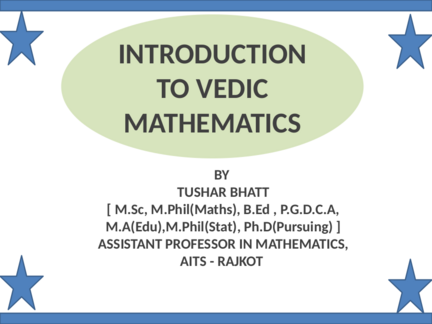# Introduction to Vedic Mathematics.Contributed by:This PPT contains The Historical Background, Basic Examples, Quiz, and a complete introduction to Vedic Mathematics.
1. INTRODUCTION
TO VEDIC
MATHEMATICS
BY
TUSHAR BHATT
[ M.Sc, M.Phil(Maths), B.Ed , P.G.D.C.A,
M.A(Edu),M.Phil(Stat), Ph.D(Pursuing) ]
ASSISTANT PROFESSOR IN MATHEMATICS,
AITS - RAJKOT
2. What is Vedic mathematics?
Vedic Mathematics is the surname given to a supposedly ancient
system of calculation which was "rediscovered" from the Vedas
between 1911 and 1918 by Sri Bharati Krishna Tirthaji Maharaj
(1884-1960). According to Tirthaji, all of Vedic mathematics is
based on sixteen Sutras, or word-formulae.
3. Vedic mathematics – The Historical Background
Welcome to the wonderful world of "Vedic" mathematics, a science
that its founder claims was lost due to the advent of modern
mathematics. Vedic mathematics is said by its founder to be a gift
given to this world by the ancient sages of India, though there is no
historical evidence whatsoever for this claim. It is a system for
limited arithmetic and polynomial calculation which is simpler and
more enjoyable than the equivalent algorithms of modern
Vedic Mathematics is the surname given to a supposedly ancient
system of calculation which was "rediscovered" from the Vedas
between 1911 and 1918 by Sri Bharati Krishna Tirthaji Maharaj
(1884-1960). According to Tirthaji, all of Vedic mathematics is based
on sixteen Sutras, or word-formulae. For example, "Vertically and
Crosswise" is one of these Sutras. These formulae are intended to
describe the way the mind naturally works, and are therefore
supposed to be a great help in directing the student to the
appropriate method of solution.
4. Vedic mathematics – The Historical Background
5. Vedic mathematics – The Historical Background
Perhaps the most striking feature of the Tirthaji system is
its coherence. The whole system is interrelated and
unified: the general multiplication method, for example,
is easily reversed to allow one-line divisions, and the
simple squaring method can be reversed to give one-line
square roots. And, these are all easily understood. This
unifying quality is very satisfying, it makes arithmetic easy
and enjoyable, and it encourages innovation.
6. Vedic mathematics
7. Vedic mathematics
8. Vedic mathematics
9. Vedic mathematics
10.
11.
12.
13.
14.
15.
16.
17.
18.
19.
20.
21.
22.
23.
24.
25.
26.
27.
28.
29. Exercise
1) 89 * 56 = ___________________________
2) 49*89 = ____________________________
3) 88*92 = ____________________________
4) 59*68 = ____________________________
30. SOLUTION
1) 89*56
89 89-100 = -11
56 56-100 = -44
(89+56)-100 -11* -44 = 484
45 484
4 5+4 = 9 84
4984
31. SOLUTION
2) 49*89
49 49-100 = -51
89 89-100 = -11
(49+89)-100 -51* -11 = 561
38 561
3 8+5 = 13 61
61
3+1=4 3
4361
32. SOLUTION
3) 88*92
88 88-100 = -12
92 92-100 = -8
(88+92)-100 -12* -8 = 96
80 96
8096
33. SOLUTION
4) 59*68
59 59-100 = -41
68 68-100 = -32
(59+68)-100 -41* -32 = 1312
27 1312
- 27+13 12
- 40 12
4012
34.